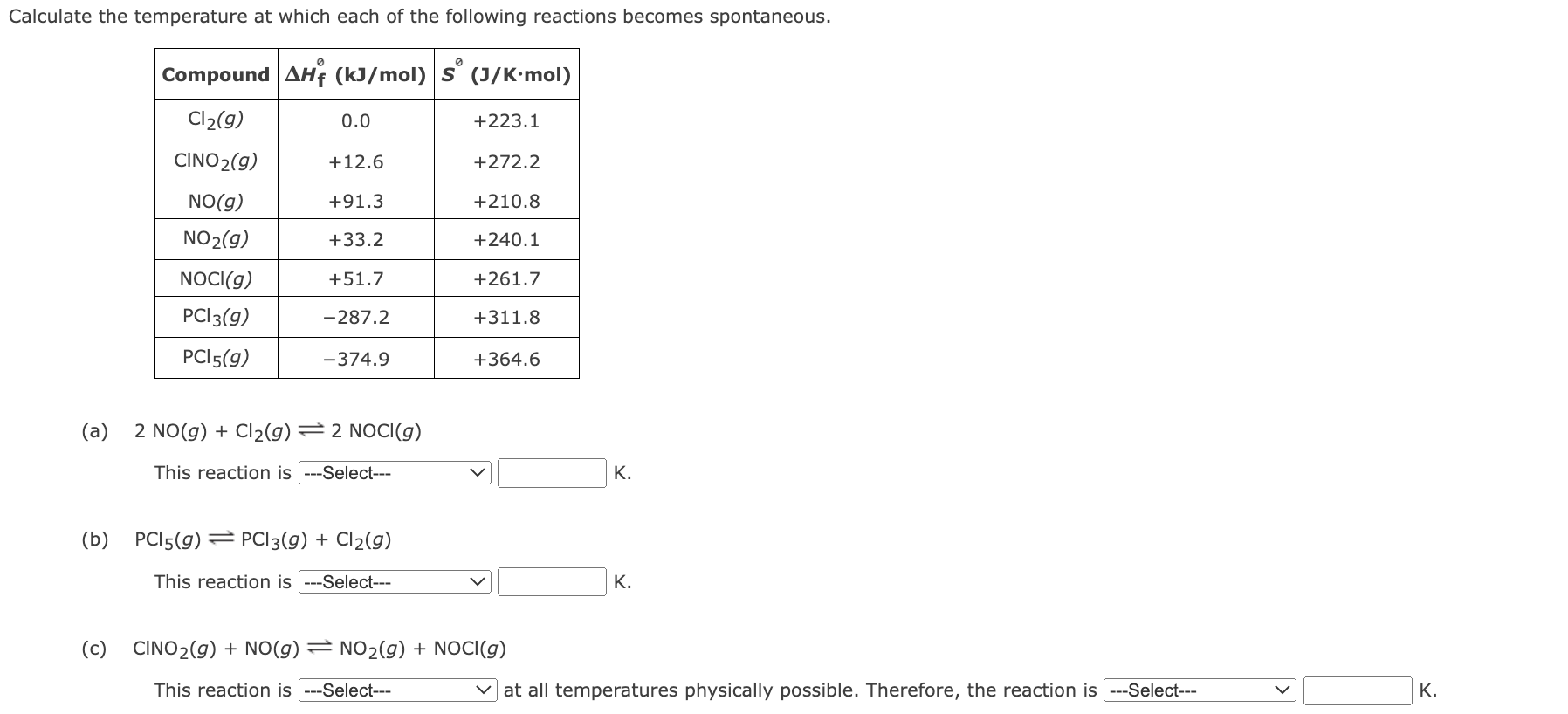# (Solved): Please help me ow to get to a T? For the first one I had: Hrxn = 2 Hf(NOCl) [2H ...

For the first one I had:

ΔHrxn  = 2 ΔHf⁰(NOCl) – [2ΔHf⁰(NO)+ ΔHf⁰Cl2] = 2*51.7 kJ/mol – [2*91.3 kJ/mol +0 kJ/mol] = -79.2 kJ= -79200J

ΔSrxn = 2 ΔS⁰(NOCl) – [2ΔS⁰(NO)+ ΔS⁰Cl2] =2*261.7 J/K·mol – 2*210.8 J/K·mol-223.1 J/K·mol = -121.3J/K

- How do I get a ΔT?

-Also, what does it mean:

The reaction is spontaneous above or spontaneous below?Calculate the temperature at which each of the following reactions becomes spontaneous. (a) This reaction is K. (b) This reaction is K. (c)

We have an Answer from Expert

Solution =

Temperature   find in the calculation means temperature at equilibrium

At equilibrium temperature   .the reaction is spontaneous if temperature   is greater than

We have an Answer from Expert